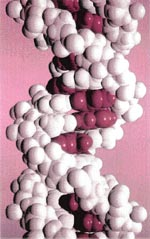# Final solutions

Scientists are using the DNA as a computer to solve complex problems

###### Published: Sunday 30 April 2000the genetic material in our cells is a wonder of nature. By using a few building blocks, nature has been able to encode a vast amount of information in our DNA (deoxyribonucleic acid) about us. Now a team of scientists at the University of Wisconsin have used DNA as a computer to get some clues about one of the most prominent unsolved problems in computer science: the so-called travelling salesman problem.

There are some problems in computer science which are considered to be so hard that even the fastest and most powerful computer takes a long time to solve them. One such problem is the travelling salesman problem. A travelling salesman has to visit a number of cities. The problem amounts to finding the shortest possible route for him to take. The problem might sound trivial, but as the number of cities increases, the complexity of the problem and hence its solution increases enormously.The problem is encountered in many different areas in science and operations research and belongs to a class of problems called np complete. For instance, molecular biologists wanting to find the most stable shape of a long protein molecule face the same kind of problem.

Just about the only way to solve such problems is to try out all possible solutions and this is precisely what makes the problem intractable in realistic cases where the number of solutions is very large. Lloyd Smith and colleagues have used dna to sort out the correct solution from amongst the possible solutions. The reason why dna "computing" is able to do what most computers find hard is that the dna computer goes through all the solutions in parallel, thus saving enormous amount of computing time.

A strand of dna encodes the information needed to put together protein molecules in a string of four different molecular units. This data-bearing capability of dna is the basis of a new type of computer. Tailor-made lengths of dna that encode all possible solutions to a particular problem can allow researchers to weed out the 'wrong answers'.

Instead of working on a np complete problem, the group worked on a related problem called the satisfiabilty problem or sat . In such a problem, the solution has to satisfy many criteria simultaneously. The one that they chose had 16 possible solutions, all of which were encoded, as a strand of dna. Then using chemical techniques to sift out the "wrong" strands, the researchers were able to get the dna that encoded the correct answer - the solution that met all the criteria for the solution. Though this method cannot be scaled up to solve real life problems like the one encountered in airline scheduling for instance, it still demonstrates that in certain cases chemical molecules might be smarter and faster than microprocessors (Nature , Vol 403, p175, January 2000).